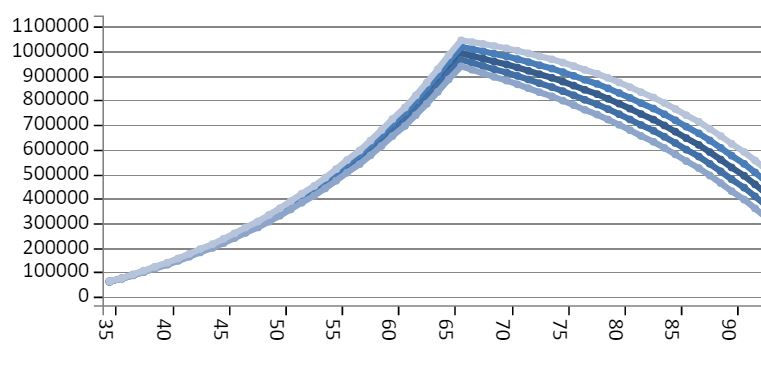top of page

# Volatility Primer 1: Volatility Background

Volatility is a statistical measure of the variability of returns in a given asset or index. Volatility of returns is extremely important in calculating the probability of a future value of a portfolio, such as a retirement portfolio, which is used to generate an income stream. For example, when using a specific return, without factoring the affects of volatility, a retirement account may have an exact 100% chance of meeting a specific goal. Factoring the effects of volatility, or variation in returns, immediately reduces the probability of meeting that goal to 50% since returns have an equal probability of being above or below the average return.

The simplest way to show the effects of volatility is to plot possible portfolio values in a hypothetical retirement account for different levels of volatility using the ProFolio Retirement Calculator.

Each of the retirement accounts have an initial starting value of \$50,000 earning an annual return of 5% with an annual contribution of \$10,000 until retirement age. Withdrawals from the portfolio consist of \$60,000 beginning at the retirement age of 67. The only difference in each of the graphs is the amount of volatility.

Each graph contains 5 lines. The middle line is the mean return. The portfolio return has a 50% probability of being above this value and a 50% probability of being below this value regardless of the volatility. The other lines above and below the mean return are equal probability lines (plus and minus one and two standard deviations from the mean).

Here is the plot of different possible portfolio values with 0% volatility. Cash has 0% volatility.Below is the plot of different possible portfolio values with 1% volatility. 90-day T-Bills have a volatility of around 1%.Next is the plot of different possible portfolio values with 10% volatility. A diversified portfolio of stocks and bonds has a volatility of around 10%.Here is the plot of different possible portfolio values with 30% volatility. Individual stocks can have volatilities of around 30% or even higher.The mean value of all these plots show a portfolio value close to one million dollars at retirement. However, the possible portfolio values vary dramatically as the volatility increases. The income stream the portfolios generate in retirement become less and less dependable with increasing volatility until there is little confidence that they can be relied on.

In order to be confident in your retirement plan you need to have confidence in both the expected return as well as the expected volatility.

Disclosure:

The information presented here is the opinion of the author and may quickly become outdated and is subject to change without notice. All material presented in this article are compiled from sources believed to be reliable, however accuracy cannot be guaranteed. No person should make an investment decision in reliance on the information presented here.

The information presented here is distributed for education purposes only and is not an offer to buy or sell or a solicitation of an offer to buy or sell any security or participate in any particular trading strategy.

Performance data showing past performance results is no guarantee of future returns.